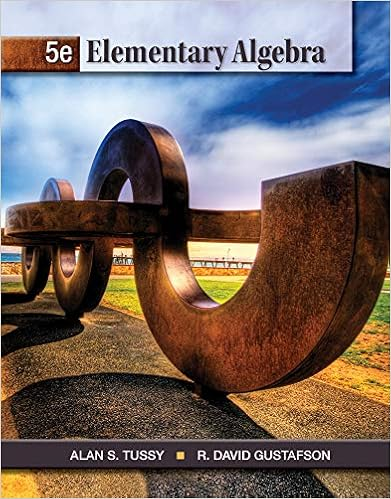# Lecture Notes 10 - Function Operations and Inverses The...

• Notes
• HighnessDangerFish3844
• 10

This preview shows page 1 - 3 out of 10 pages.

##### We have textbook solutions for you!
The document you are viewing contains questions related to this textbook.The document you are viewing contains questions related to this textbook.
Chapter 1 / Exercise 5
Elementary Algebra
Tussy/GustafsonExpert Verified
1
0
-1
1
9
9
9
##### We have textbook solutions for you!
The document you are viewing contains questions related to this textbook.The document you are viewing contains questions related to this textbook.
Chapter 1 / Exercise 5
Elementary Algebra
Tussy/GustafsonExpert Verified
2g of()0( )=g(f(0)) = g 02()=g 0( )=0 – 3 =- 3g of()3( )=g(f(3)) = g 32()=g 9( )=9 – 3 =6g of()-1()=g(f(-1)) = g-1()2()=g 1( )=1 – 3 =- 2, and, in general,g of()x( )=g(f(x)) = g x2()=x2-3Notice that, for these two functions f and g, g of()x( )f og()x( )for any x value we tried,and they certainly are not generally equivalent. There is a value for whichg of()x( )=f og()x( ). Check out x = 2. You will find that g of()2( )=f og()2
The following examples should help.(2) For the two functions given above [f(x) = x + 1 and g(x) = 2x – 1],fog()x( )=f g x( )()= f2x -1()=2x -1()+1 =We’re basically substituting the formula for g(x), and then applying the rule for f on theresult.(3) Let f x( )=x2and g x( )=x – 3. Find f og()0( ), f og()3( ), f og()-1(), and f og()f og()0( )=f(g(0)) = f 0 – 3()=f-3()=-3()2=9f og()3( )=f(g(3)) = f 3 – 3()=f 0( )=0( )2=0f og()-1()=f(g(-1)) = f -1 – 3()=f-4()=-4()2=16, and, in general,f og()x( )=f(g(x)) = f x – 3()=x -3()2=x – 3()x – 3()=x2-6x +As with subtraction and division of functions, the order of composition is very important. Thenext example is chosen to illustrate this fact.(4) Let f x( )=x2and g x( )=x – 3. Find g of()0( ), g of()3( ), g of()-1(), and g of()2xx( ).,,9.x( ).
,
,
.
( ).
•••